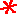Main      Site Guide### 6×6 Logi-Number Puzzles

Logi-Number Puzzles are a cross between logic problems and mathematical puzzles. In each, you must determine what values the variables are equal to, using the rules of the game and the given clues. The rules are: (1) all the variables are equal to integer values between one and the number of variables in the puzzle, and (2) none of the variables are equal to each other. For example, if there are six variables, each will equal a number from 1 to 6. Since no variable equals another, all six values will be used.

You may find it convenient to solve the puzzles with the aid of the grids provided. Click on a square to turn it red when you know something to be false (for example, if you know that A is not equal to 3, turn box A3 red); click on a square a second time to turn it green to denote something that's true. (Clicking a third time turns the box white again.) Clicking on a clue will turn it gray; this is useful when you are finished with a clue. If you would prefer to solve these puzzles on paper, simply print them out and use a pencil to fill in the grid.

## #411. F + E = C
2. C + A = F + D
3. E + B = C + D
4. D + A = C
1 2 3 4 5 6
A
B
C
D
E
F

Hint

Solution

## #421. C > B
2. E - B = F - 3
3. A + C = D
4. C + B = E + A
1 2 3 4 5 6
A
B
C
D
E
F

Hint

Solution

1. B + C = 5
2. B + E = C
3. A + B = F
4. C + B = D
1 2 3 4 5 6
A
B
C
D
E
F

Hint

Solution

## #44

1. A > C
2. A + F = D
3. D ≠ 6
4. C + A = B
5. C + E = A + F
1 2 3 4 5 6
A
B
C
D
E
F

Hint

Solution

1. A + C = 8
2. F - 1 = D
3. 2A = E + 4
1 2 3 4 5 6
A
B
C
D
E
F

Hint

Solution

## #46

1. F + B = C
2. E + F = B + D
3. E + 3 = C
4. A + 1 = C
1 2 3 4 5 6
A
B
C
D
E
F

Hint

Solution

1. 4E = D + 19
2. 2A = D + 3
3. B + A = C
1 2 3 4 5 6
A
B
C
D
E
F

Hint

Solution

## #48

1. 3E = C + 7
2. E + C = B + F
3. F + D = A
1 2 3 4 5 6
A
B
C
D
E
F

Hint

Solution

## #49

1. B + A = E
2. A + C = B + E
3. C + E = D
1 2 3 4 5 6
A
B
C
D
E
F

Hint

Solution

1. C < B
2. A ≠ 5
3. 2D = B + 5
4. F + D = E
1 2 3 4 5 6
A
B
C
D
E
F

Hint

Solution